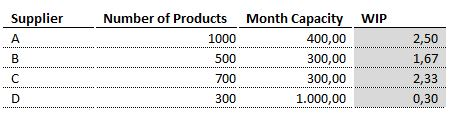New to QlikView

Discussion board where members can get started with QlikView.

Announcements
QlikWorld 2020: Join us May 11 - 14, 2020 in Phoenix, AZ. Register early and save \$400. Learn More
Highlighted
New Contributor III

Calculating weighted number in an expression

Dear All,

I am working on a qlikview application which aims to calculate the aggregate work in progress `(WIP) in our suppliers. The calculus of their work in progress is the Number of Products already demanded (column 2) divided by the supplier´s month capacity (column 3).

However, we want also to calculate the weighted WIP (WWIP), which is done by the sum of each supplier´s WIP times it´s number of products divided by the total number of products.

In the case expressed by the table below, we have the following calculation:

1000*2,5 + 500 * 1,67 + 700* 2,33 + 300*0,3 / (1000+500+700+300) = 2,02Can anyone help me doing the WWIP in a single expression?

Regards,

Tags (3)
4 RepliesMVP

Re: Calculating weighted number in an expression

May be this?

((Sum({<Supplier = {A}>} [Number of Products]) * Sum({<Supplier = {A}>}WIP)) +

(Sum({<Supplier = {B}>} [Number of Products]) * Sum({<Supplier = {B}>}WIP)) +

(Sum({<Supplier = {C}>} [Number of Products]) * Sum({<Supplier = {C}>}WIP)) +

(Sum({<Supplier = {D}>} [Number of Products]) * Sum({<Supplier = {D}>}WIP))) / Sum(TOTAL [Number of Products])

Before develop something, think If placed (The Right information | To the right people | At the Right time | In the Right place | With the Right context)MVP

Re: Calculating weighted number in an expression

What is expression for "Number of Product"?MVP

Re: Calculating weighted number in an expression

You need WWIP in a KPI or text object? May be try like this

Sum(Aggr((Expression4NumberOfProduct * Expression4WIP)/Expression4NumberOfProductWithTOTALQualifier, Supplier))MVP

Re: Calculating weighted number in an expression

sum(aggr((Expression of Number of Product)*sum(distinct Weight),Supplier))/(Expression of Number of Product)